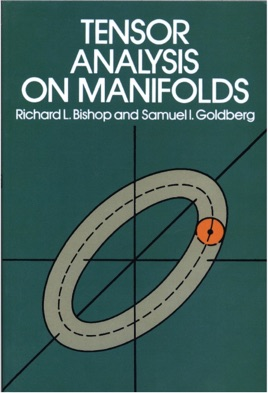• 9,99 €

## Descripción de la editorial

"This is a first-rate book and deserves to be widely read." — American Mathematical Monthly
Despite its success as a mathematical tool in the general theory of relativity and its adaptability to a wide range of mathematical and physical problems, tensor analysis has always had a rather restricted level of use, with an emphasis on notation and the manipulation of indices. This book is an attempt to broaden this point of view at the stage where the student first encounters the subject. The authors have treated tensor analysis as a continuation of advanced calculus, striking just the right balance between the formal and abstract approaches to the subject.
The material proceeds from the general to the special. An introductory chapter establishes notation and explains various topics in set theory and topology. Chapters 1 and 2 develop tensor analysis in its function-theoretical and algebraic aspects, respectively. The next two chapters take up vector analysis on manifolds and integration theory. In the last two chapters (5 and 6) several important special structures are studied, those in Chapter 6 illustrating how the previous material can be adapted to clarify the ideas of classical mechanics. The text as a whole offers numerous examples and problems.
A student with a background of advanced calculus and elementary differential equation could readily undertake the study of this book. The more mature the reader is in terms of other mathematical knowledge and experience, the more he will learn from this presentation.